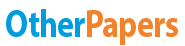# Preference Relations and Utility Theory

Essay by   •  June 9, 2011  •  Essay  •  298 Words (2 Pages)  •  1,625 Views

## Essay Preview: Preference Relations and Utility Theory

Report this essay
Page 1 of 2

Previously Gerard Debreu discussed Preference Relations and Utility Theory and now answers the question "When can we get a continuous utility function?"

He begins by showing how a concave utility function has convex indifference curves. He gives the following definition:

If you take a convex set X and take the graph of the utility function, therefore you need one more dimension, then the set of points below the graph is a convex set.

This means that if you take any point in R2 (above) any points to the right of IC1 is going to be a convex set. This can also be applied more generally to any indifference surface in Rn+.

If you take the above definition of a concave utility function together with the assumptions of continuity, reflexivity and transitivity, it is still not sufficient for a preference relation to be concave.

For example, with a single good an individual can reach a satiated point. Therefore with the addition of monotone preference relation on X, there is at least one other good that will be welfare improving and then the preference relation is locally nonsatiated.

The concept of indifference curves and utility functions cannot be observed, and therefore cannot be tested. Although the consequences of this concept can be observed, that is, the demand behaviour. Revealed Preference Theory begins to reconcile this problem and here the proposition is taken directly from the text:

The basic setup of the model is that a consumer has a finite commodity space, prices are fixed and positive and determine the 'competitive' budget, and the consumer cannot spend more than their budget.

1. The problem of rationality

The observable demand is not from a unique preference relation so therefore it is not possible to "...find the preference generating choices, but only some preferences - a set of 'equivalent' preferences".

...

...